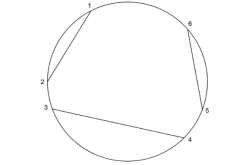# Count ways to divide circle using N non-intersecting chords in C++

Given an integer N as input for a number of chords in a circle with 2*N end points. The goal is to count the ways in which we can divide that circle using such chords so that no chord intersects with each other.

For N=3, points will be 6, 1 way of getting 3 chords is between 1−2, 3−4, 5−6Other ways −

1−6, 2−5, 3−4
1−2, 3−6, 4−5
1−4, 2−3, 5−6
1−6, 2−3, 4−5

Total 5 ways.

For Example

## Input

N=4

## Output

Count of ways to divide circle using N non-intersecting chords are: 14

## Explanation

There will be a total 8 points between which we can draw chords. After
drawing the first chord, the rest of the points will be divided into two sets. No chord can be drawn between points from set 1 and set 2 as they will intersect with the first chord.

## Input

N=6

## Output

Count of ways to divide circle using N non−intersecting chords are: 132

## Explanation

There will be a total 12 points between which we can draw chords. After
drawing the first chord, the rest of the points will be divided into two sets. No chord can be drawn between points from set 1 and set 2 as they will intersect with the first chord.

Approach used in the below program is as follows

In this approach we will count ways using previous counts. If we draw any chord between 2 points, the rest of the points will be divided into two sets set1 and set2, if we draw any chord between points in these two sets then they will intersect with the first chord.

• Take an integer N as input.

• Function divide_circle(int N) takes number and returns the count of ways to divide circle using N non−intersecting chords

• Total number of points will be 2*N as total_points.

• Take an array total_cuts[] storing the counts of ways.

• For 0 or 2 points there will be only 1 way to initialize total_cuts, total_cuts with 1.

• For all other points starting from points=4, total ways will be ways with points i and rest n−i−1 points.

• So take total_cuts[i] += (total_cuts[j] * total_cuts[i−2−j])

• At the end we have total_cuts[ total_points ] as total number of ways.

• Return total_cuts[ total_points ] as result at the end of the loop.

## Example

Live Demo

#include <bits/stdc++.h>
using namespace std;
int divide_circle(int N){
int total_points = 2 * N;
int total_cuts[total_points + 1] = { 0 };
total_cuts = 1;
total_cuts = 1;
for (int i = 4; i <= total_points; i += 2){
for (int j = 0; j < i−1; j += 2){
total_cuts[i] += (total_cuts[j] * total_cuts[i−2−j]);
}
}
}
int main(){
int N = 3;
cout<<"Count of ways to divide circle using N non−intersecting chords are:"<<divide_circle(N);
return 0;
}

## Output

If we run the above code it will generate the following output −

Count of ways to divide circle using N non-intersecting chords are: 5